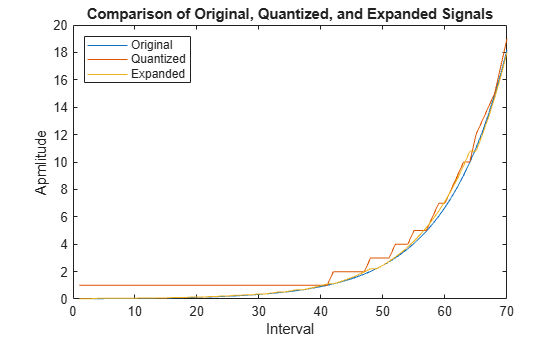# compand

Source coding mu-law or A-law compressor or expander

## Syntax

``out = compand(in,param,v)``
``out = compand(in,param,v,method)``

## Description

example

````out = compand(in,param,v)` performs mu-law compression on the input data sequence. The `param` input specifies the mu-law compression value and must be set to a mu value for mu-law compressor computation (a mu-law value of 255 is used in practice). `v` specifies the peak magnitude of the input data sequence.```

example

````out = compand(in,param,v,method)` performs mu-law or A-law compression or expansion on the input data sequence. `param` specifies the mu-law compander or A-law compander value (a mu-law value of 255 and an A-law value of 87.6 are used in practice). `method` specifies the type of compressor or expander computation for the function to perform on the input data sequence.```

## Examples

collapse all

Generate a data sequence.

`data = 2:2:12`
```data = 1×6 2 4 6 8 10 12 ```

Compress the data sequence by using a mu-law compressor. Set the value for mu to 255. The compressed data sequence now ranges between 8.1 and 12.

`compressed = compand(data,255,max(data),'mu/compressor')`
```compressed = 1×6 8.1644 9.6394 10.5084 11.1268 11.6071 12.0000 ```

Expand the compressed data sequence by using a mu-law expander. The expanded data sequence is nearly identical to the original data sequence.

`expanded = compand(compressed,255,max(data),'mu/expander')`
```expanded = 1×6 2.0000 4.0000 6.0000 8.0000 10.0000 12.0000 ```

Calculate the difference between the original data sequence and the expanded sequence.

`diffvalue = expanded - data`
```diffvalue = 1×6 10-14 × -0.0444 0.1776 0.0888 0.1776 0.1776 -0.3553 ```

Generate a data sequence.

`data = 1:5;`

Compress the data sequence by using an A-law compressor. Set the value for A to 87.6. The compressed data sequence now ranges between 3.5 and 5.

`compressed = compand(data,87.6,max(data),'A/compressor')`
```compressed = 1×5 3.5296 4.1629 4.5333 4.7961 5.0000 ```

Expand the compressed data sequence by using an A-law expander. The expanded data sequence is nearly identical to the original data sequence.

`expanded = compand(compressed,87.6,max(data),'A/expander')`
```expanded = 1×5 1.0000 2.0000 3.0000 4.0000 5.0000 ```

Calculate the difference between the original data sequence and the expanded sequence.

`diffvalue = expanded - data`
```diffvalue = 1×5 10-14 × 0 0 0.1332 0.0888 0.0888 ```

When transmitting signals with a high dynamic range, quantization using equal length intervals can result in loss of precision and signal distortion. Companding is a operation that applies a logarithmic computation to compress the signal before quantization on the transmit side and applies an inverse operation to expand the signal to restore it to full scale on the receive side. Companding avoids signal distortion without the need to specify many quantization levels. Compare distortion when using 6-bit quantization on an exponential signal with and without companding. Plot the original exponential signal, the quantized signal and the expanded signal.

Create an exponential signal and calculate its maximum value.

```sig = exp(-4:0.1:4); V = max(sig);```

Quantize the signal by using equal-length intervals. Set partition and codebook values, assuming 6-bit quantization. Calculate the mean square distortion.

```partition = 0:2^6 - 1; codebook = 0:2^6; [~,qsig,distortion] = quantiz(sig,partition,codebook);```

Compress the signal by using the `compand` function configured to apply the mu-law method. Apply quantization and expand the quantized signal. Calculate the mean square distortion of the companded signal.

```mu = 255; % mu-law parameter csig_compressed = compand(sig,mu,V,'mu/compressor'); [~,quants] = quantiz(csig_compressed,partition,codebook); csig_expanded = compand(quants,mu,max(quants),'mu/expander'); distortion2 = sum((csig_expanded - sig).^2)/length(sig);```

Compare the mean square distortion for quantization versus combined companding and quantization. The distortion for the companded and quantized signal is an order of magnitude lower than the distortion of the quantized signal. Equal-length intervals are well suited to the logarithm of an exponential signal but not well suited to an exponential signal itself.

`[distortion, distortion2]`
```ans = 1×2 0.5348 0.0397 ```

Plot the original exponential signal, the quantized signal, and the expanded signal. Zoom in on axis to highlight the quantized signal error at lower signal levels.

```plot([sig' qsig' csig_expanded']); title('Comparison Between Original, Quantized, and Expanded Signals'); xlabel('Interval'); ylabel('Apmlitude'); legend('Original','Quantized','Expanded','location','nw'); axis([0 70 0 20])```## Input Arguments

collapse all

Input data sequence, specified as a row vector. This input specifies the data sequence for the function to perform compression or expansion.

Data Types: `double`

mu or A value of the compander, specified as a positive scalar. The prevailing values used in practice are µ = `255` and A = `87.6`.

Data Types: `double`

Type of compressor or expander computation for the function to perform on the input data sequence, specified as one of these values.

• `mu/compressor`

• `mu/expander`

• `A/compressor`

• `A/expander`

Data Types: `char` | `string`

Peak magnitude of the input data sequence, specified as a positive scalar.

Data Types: `double`

## Output Arguments

collapse all

Compressed or expanded signal, returned as a positive row vector. The size of `out` matches that of input argument `in`.

## Algorithms

In certain applications, such as speech processing, using a logarithmic computation (called a compressor) before quantizing the input data is common. The inverse operation of a compressor is called an expander. The combination of a compressor and expander is called a compander.

For a given signal, x, the output of the (µ-law) compressor is

`$y=\frac{\mathrm{log}\left(1+\mu |x|\right)}{\mathrm{log}\left(1+\mu \right)}\mathrm{sgn}\left(x\right).$`

µ is the µ-law parameter of the compander, `log` is the natural logarithm, and `sgn` is the signum function (`sign` in MATLAB®).

µ-law expansion for input signal x is given by the inverse function y-1,

For a given signal, x, the output of the (A-law) compressor is

A is the A-law parameter of the compander, `log` is the natural logarithm, and `sgn` is the signum function (`sign` in MATLAB).

A-law expansion for input signal x is given by the inverse function y-1,

 Sklar, Bernard. Digital Communications: Fundamentals and Applications. Englewood Cliffs, NJ: Prentice-Hall, 1988.